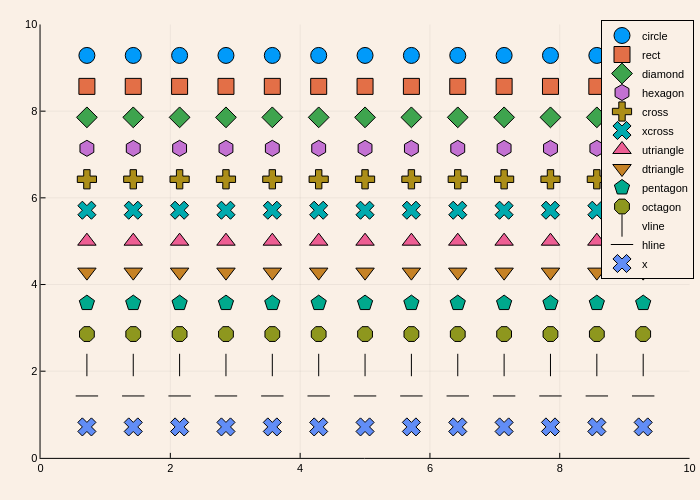# Marker typesusing Plots
plotlyjs()
markers = filter((m->begin
m in Plots.supported_markers()
end), Plots._shape_keys)
markers = permutedims(markers)
n = length(markers)
x = (range(0, stop = 10, length = n + 2))[2:end - 1]
y = repeat(reshape(reverse(x), 1, :), n, 1)
scatter(x, y, m = markers, markersize = 8, lab = map(string, markers), bg = :linen, xlim = (0, 10), ylim = (0, 10))